# The tourist

The tourist walked a quarter of the way on the first day, a third of the rest on the second day, and 20 km on the last day. How many km did he walk in three days?

x =  40

### Step-by-step explanation:

x/4+(x-x/4)/3+20=x

6x = 240

x = 40

Our simple equation calculator calculates it.Did you find an error or inaccuracy? Feel free to write us. Thank you!Alpha_shi_chan
ReallyDr Math
I verified, solution is correct to this questionTips to related online calculators
Need help to calculate sum, simplify or multiply fractions? Try our fraction calculator.
Do you have a linear equation or system of equations and looking for its solution? Or do you have a quadratic equation?
Do you want to convert length units?
Do you want to convert time units like minutes to seconds?

## Related math problems and questions:

• Three days way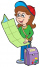The tourist walked a quarter of the way on the first day, a third of the rest on the second day and 20 km on the last day. How many km did he cover in three days?
• The touristThe tourist traveled 78km in 3 hours. Part of the journey went on foot at 6km/h, the rest of the trip by bus at 30km/h. How long did he walk?
• The hikerThe hiker will travel 40% of the route on the first day 1and/3 of the rest of the second day. Last day 30 km. What was the length of the 3-day trip? How many kilometers did he walk each day?
• The tourist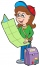The tourist has walked 3/4 of the route. The destination is 12.5 km away. How many kilometers did the route measure?
• Hiker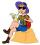Hiker went half of the trip the first day, a third of the trip the second day, and remains 15 km. How long the trip he planned?
• The touristThe tourist wanted to walk the route 16 km at a specific time. He, therefore, came out at the necessary constant speed. However, after a 4 km walk, he fell unplanned into the lake, where he almost drowned. It took him 20 minutes to get to the shore and re
• TruckThe truck passed 4550 km in 5 days. The first three days passed every day the same way. The fourth day passed 630 km and the fifth day was 920 km. How many km has passed the first three days?
• The ship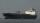The ship went upstream speed of 20 km/h in relation to water. River flows at speed 10 km/h. After half an hour, he stopped and returned downstream to the starting point. How long it took way back when even if downstream speed of ship is 20 km/h in relatio
• Average speed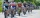The average speed of a pedestrian who walked 10 km was 5km/h, the average speed of a cyclist on the same track was 20km/h. In how many minutes did the route take more than a cyclist? Q
• Three-day tripThe cyclist on three-day trip travel 30% of the total route on the first day, 3/5 of the rest on the second day and 35 km on the third day. How many kilometers did travel cyclists each day and how many?
• WayIf the current way to school was 2 km shorter then if Milan swipe one half he will have already one quarter of the way to school behind. How long is Milan's way to school?
• Scouts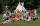The boys from scout group traveled 5 days distance 115 km. Every day walked 1.5 km less than the previous day. How many kilometers scouts walked in the first day?
• The cyclistThe cyclist drove from A to B, 60 km away. The first half drove at 30km/h, the second at 20km/h. How long did it take for him?
• Tourist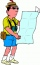A tourist walked an average speed of 3.5 km/h route in 6 hours. Calculate how many hours he would have passed at an average speed of 5.5 km/h.
• Cyclist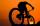Cyclist rode two-fifths of the way the first day. The second day drove 5 km less while traveled three-eighths of route. How many kilometers does he have to finish target?
• Scouts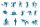Three scouts went on a three day trip. The second day went 4 km more than the first day. The third day went two times less than the first day. They went along 54 km. How many kilometers went every day together?
• The creek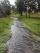Workers cleaned the creek within 3 days. The first day cleanse one-fifth the creek's length, the next day 40% of the stream's length, and the third day 8 km length of the creek. How long is a creek?